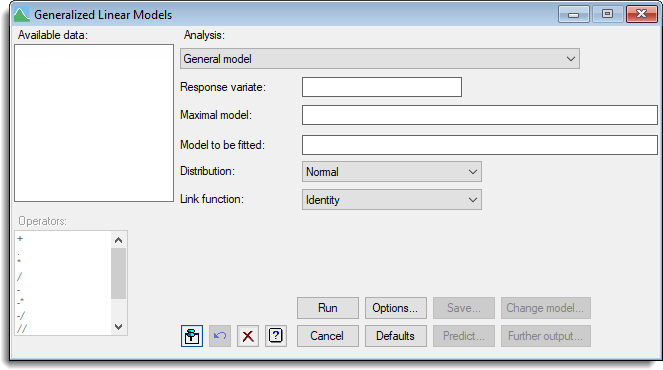1. Home
2. Generalized Linear Models

# Generalized Linear Models

Generalized Linear Models (or GLMs) extend the ordinary regression framework to situations where the observations of the response variate do not follow a Normal distribution, or where a transformation needs to be applied before a linear model can be fitted. The Generalized Linear Models dialog can be used to fit a range of models including log-linear models and logistic regression. In addition there are menus for ordinal regression and all subset regression.

Stats | Regression Analysis | Generalized Linear Models.
2. Fill in the fields as required then click Run.

You can set additional Options then after running, you can save the results by clicking Save.## Generalized linear models dialog

The following range of models can be fitted using the Generalized Linear Models dialog. Note that the General Model can be used a fit a wide range of models by specifying a model formula, distribution and link function.